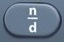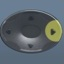# Triangle

Triangle = Flat Shape with Three SidesBasics
s1 = side 1
s2 = side 2
s3 = side 3
x = angle x
y = angle y
z = angle z
h = height
b = base
P = Perimeter = distance along sides of triangle
A = Area = area covering surface of triangle (measured in square units)

The three angles (x, y, z) inside the triangle add up to 180°.

The right triangle pictured above, in which one of the angles measures 90°, is probably the most common type of triangle featured on the GED.

For more about the right triangle, see Pythagorean Theorem.

Formulas (Mathematics Formula Sheet
)
P = s1 + s2 + s3$\bf\displaystyle A=\frac{1}{2}bh$

Formulas (NOT on Mathematics Formula Sheet)

s1 = P – s2 – s3
s2 = P – s1 – s3
s3 = P – s1 – s2

b = 2 × A ÷ h
h = 2 × A ÷ b

x + y + z = 180°
x = 180° – y – z
y = 180° – x – z
z = 180° – x – z

Question
A right triangular billboard has sides of 6 feet, 8 feet, and 10 feet, respectively.  What is its perimeter?

24 feet

P = s1 + s2 + s3
P = 6 + 8 + 10 = 24

Input Display Commentblinker clears screen
6 + 8 + 10 6+8+10 P = s1 + s2 + s324 Answer
###### Triangle

Question
A right triangular billboard has a height of 6 feet and a base of 8 feet.  What is its area?

24 square feet$\bf\displaystyle A=\frac{1}{2}bh$$\bf\displaystyle A=\frac{1}{2}\times 8\times 6\$ = 24

Input Display Commentblinker clears screen12x 8 x 6$\bf\displaystyle \frac{1}{2}$*8*6$\bf\displaystyle A=\frac{1}{2}bh$24 Answer
###### Triangle

Question
A triangular piece of pizza has an angle x that measures 33° and an angle z that measures 90°.  What is the measurement of angle y (the triangular slice of pizza’s other angle) in degrees?

57°

y = 180° – x – z
y = 180 – 33 – 90 = 57

Input Display Commentblinker clears screen
180 – 33 – 90 180 – 33 – 90 y = 180° – x – z57 Answer
###### Triangle

Question
A right triangular billboard with a perimeter of 30 feet has one side that measures 5 feet and another side that measures 13 feet.  What is the length of its third side?

12 feet

s3 = P – s1 – s2
s3 = 30 – 5 – 13 = 12

Input Display Commentblinker clears screen
30 – 5 – 13 30-5-13 s3 = P – s1 – s212 Answer
###### Triangle

Question
A triangular sign has an area of 42 square feet and a height of 7 feet.  How long is its base?

12 feet

b = 2 × A ÷ h
b = 2 × 42 ÷ 7 = 12

Input Display Commentblinker clears screen
2 × 42 ÷ 7 2*42÷7 b = 2 × A ÷ h12 Answer
###### Triangle

Question
A triangular sign has an area of 96 square feet and a base of 8 feet.  How high is it?

24 feet

h = 2 × A ÷ b
h = 2 × 96 ÷ 8 = 24

Input Display Commentblinker clears screen
2 × 96 ÷ 8 2*96÷8 h = 2 × A ÷ b24 Answer
###### Triangle

Practice – Questions

1.  A right triangular sign has a height of 3 feet, base of 4 feet, and hypotenuse of 5 feet.  What is its perimeter?

2.  A right triangular sign has a height of 3 feet, base of 4 feet, and hypotenuse of 5 feet.  What is its area?

3.  A triangular piece of pie has one angle that measures 47° and a second angle that measures 68°.  What is the measurement of the pie’s third angle in degrees?

4.  A right triangular tile with a perimeter of 34 centimeters has a base of 12 centimeters and a hypotenuse of 15 centimeters.  How high is it?

5.  A triangular sign has an area of 105 square feet and a height of 15 feet.  How long it its base?